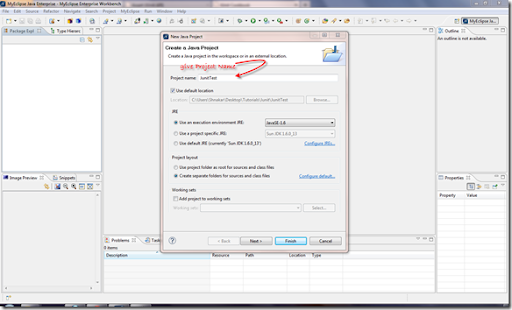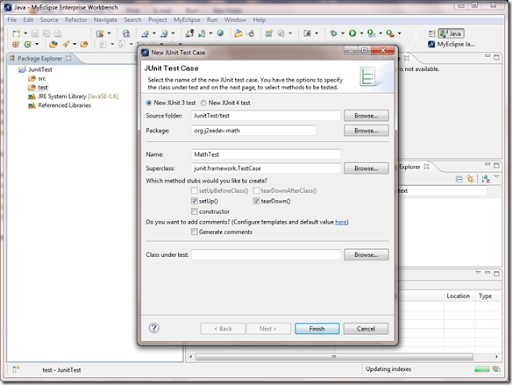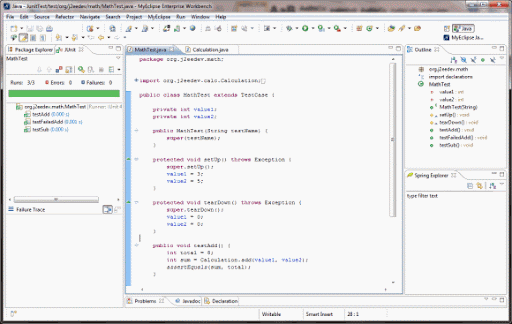{{announcement.body}}
{{announcement.title}}

# A JUnit Tutorial for Beginners

DZone 's Guide to

# A JUnit Tutorial for Beginners

· Java Zone ·
Free Resource

Comment (6)

Save
{{ articles.views | formatCount}} Views

JUnit is an open source framework designed by Kent Beck, Erich Gamma for the purpose of writing and running test cases for java programs. In the case of web applications JUnit is used to test the application with out server. This framework builds a relationship between development and testing process.

Problems that we are facing in testing the applications

If we want to test any application, In standalone, we need to call the respective method’s main() method but there are so many problems in testing the flow using main() method.

In a web application, to test the flow we need to deploy it in the server so if there is a change then again we need to restart the server. Here also facing problems while testing the application.

• Writing main method checks is convenient because all Java IDEs provide the ability to compile and run the class in the current buffer, and certainly have their place forexercising an object’s capability. There are, however, some issues with this approach that make it ineffective as a comprehensive test framework:
• There is no explicit concept of a test passing or failing. Typically, the program outputs messages simply with System.out.println; the user has to look at this and decide if it is correct.
• main has access to protected and private members and methods. While you may want to test the inner workings of a class may be desired, many tests are really about testing an object’s interface to the outside world.
• There is no mechanism to collect results in a structured fashion.
• There is no replicability. After each test run, a person has to examine and interpret the results.
The JUnit framework addresses these issues, and more
• JUnit addresses the above issues, It’s used to test an existing class. Suppose, If we have Calculation class then, we need to write CalculationTest to test the Calculation class.
• Using JUnit we can save testing time.
• In real time, in the web application development we implement JUnit test cases in the DAO classes. DAO classes can be tested with out server.
• With JUnit we can also test Struts / Spring applications but most of the time we test only DAO classes. Even some times service classes also tested using JUnit.
• Using JUnit, If we want to test the application (for web applications) then server is not required so the testing becomes fast.
Getting started with JUnit
• Extract the zip folder, you can see the documentation, and jar files for source and execution.
Creating Sample JUnit project in Eclipse / MyEclipse
• Create a Java Project (Project Name : JUnitTest)
• If you are using MyEclipse, JUnit.jar will be available with IDE itself, Right click on the project—>Properties—>Java Build Path—>Libraries—>Add Libraries—>JUnit—>Next—>Finish—>OK
• If you are Eclipse, then you need to manually add library to the buildpath. , Right click on the project—>Properties—>Java Build Path—>Libraries—>Add External Libraries—>JUnit—>Next—>Finish—>OK
• Right click on the project –>New—>Source Folder—>test
• Right click in the test –>New—>JUnit Test Case

The following screenshots will guide you through the above steps.Source Code for MathTest.java

Here is your first Debut JUnit test program.

``````package org.j2eedev.math;
/*
* @author Umashankar
* http://j2eedev.org
* Beginer JUnit Tutorial
* */

import org.j2eedev.calc.Calculation;

import junit.framework.TestCase;

public class MathTest extends TestCase {

private int value1;
private int value2;

public MathTest(String testName) {
super(testName);
}

protected void setUp() throws Exception {
super.setUp();
value1 = 3;
value2 = 5;
}

protected void tearDown() throws Exception {
super.tearDown();
value1 = 0;
value2 = 0;
}

int total = 8;
assertEquals(sum, total);
}

int total = 9;
assertNotSame(sum, total);
}
public void testSub() {
int total = 0;
int sub = Calculation.sub(4, 4);
assertEquals(sub, total);
}

}
``````

Source code for Calculation.java

``````package org.j2eedev.calc;
/*
* @author Umashankar
* http://j2eedev.org
* Beginer JUnit Tutorial
* */
public class Calculation {
public static int add(int a, int b) {
return a + b;
}
public static int sub(int a, int b) {
return a - b;
}
}``````
Method Summary
Following are the different methods available with Assert. Find the one, that suites you to test the classes in the application.
``````assertArrayEquals(byte[] expecteds, byte[] actuals)
Asserts that two byte arrays are equal.

static void
assertArrayEquals(char[] expecteds, char[] actuals)
Asserts that two char arrays are equal.

static void
assertArrayEquals(double[] expecteds, double[] actuals, double delta)
Asserts that two double arrays are equal.

static void
assertArrayEquals(float[] expecteds, float[] actuals, float delta)
Asserts that two float arrays are equal.

static void
assertArrayEquals(int[] expecteds, int[] actuals)
Asserts that two int arrays are equal.

static void
assertArrayEquals(long[] expecteds, long[] actuals)
Asserts that two long arrays are equal.

static void
assertArrayEquals(Object[] expecteds, Object[] actuals)
Asserts that two object arrays are equal.

static void
assertArrayEquals(short[] expecteds, short[] actuals)
Asserts that two short arrays are equal.

static void
assertArrayEquals(String message, byte[] expecteds, byte[] actuals)
Asserts that two byte arrays are equal.

static void
assertArrayEquals(String message, char[] expecteds, char[] actuals)
Asserts that two char arrays are equal.

static void
assertArrayEquals(String message, double[] expecteds, double[] actuals, double delta)
Asserts that two double arrays are equal.

static void
assertArrayEquals(String message, float[] expecteds, float[] actuals, float delta)
Asserts that two float arrays are equal.

static void
assertArrayEquals(String message, int[] expecteds, int[] actuals)
Asserts that two int arrays are equal.

static void
assertArrayEquals(String message, long[] expecteds, long[] actuals)
Asserts that two long arrays are equal.

static void
assertArrayEquals(String message, Object[] expecteds, Object[] actuals)
Asserts that two object arrays are equal.

static void
assertArrayEquals(String message, short[] expecteds, short[] actuals)
Asserts that two short arrays are equal.

static void
assertEquals(double expected, double actual)
Deprecated. Use assertEquals(double expected, double actual, double epsilon) instead

static void
assertEquals(double expected, double actual, double delta)
Asserts that two doubles or floats are equal to within a positive delta.

static void
assertEquals(long expected, long actual)
Asserts that two longs are equal.

static void
assertEquals(Object[] expecteds, Object[] actuals)
Deprecated. use assertArrayEquals

static void
assertEquals(Object expected, Object actual)
Asserts that two objects are equal.

static void
assertEquals(String message, double expected, double actual)
Deprecated. Use assertEquals(String message, double expected, double actual, double epsilon) instead

static void
assertEquals(String message, double expected, double actual, double delta)
Asserts that two doubles or floats are equal to within a positive delta.

static void
assertEquals(String message, long expected, long actual)
Asserts that two longs are equal.

static void
assertEquals(String message, Object[] expecteds, Object[] actuals)
Deprecated. use assertArrayEquals

static void
assertEquals(String message, Object expected, Object actual)
Asserts that two objects are equal.

static void
assertFalse(boolean condition)
Asserts that a condition is false.

static void
assertFalse(String message, boolean condition)
Asserts that a condition is false.

static void
assertNotNull(Object object)
Asserts that an object isn't null.

static void
assertNotNull(String message, Object object)
Asserts that an object isn't null.

static void
assertNotSame(Object unexpected, Object actual)
Asserts that two objects do not refer to the same object.

static void
assertNotSame(String message, Object unexpected, Object actual)
Asserts that two objects do not refer to the same object.

static void
assertNull(Object object)
Asserts that an object is null.

static void
assertNull(String message, Object object)
Asserts that an object is null.

static void
assertSame(Object expected, Object actual)
Asserts that two objects refer to the same object.

static void
assertSame(String message, Object expected, Object actual)
Asserts that two objects refer to the same object.

static <T> void

assertThat(String reason, T actual, Matcher<T> matcher)
Asserts that actual satisfies the condition specified by matcher.

static <T> void

assertThat(T actual, Matcher<T> matcher)
Asserts that actual satisfies the condition specified by matcher.

static void
assertTrue(boolean condition)
Asserts that a condition is true.

static void
assertTrue(String message, boolean condition)
Asserts that a condition is true.

static void
fail()
Fails a test with no message.

static void
fail(String message)
Fails a test with the given message.
``````
Explanation of Above application
• A test case is used to run multiple tests.

To Define a Test case:

• Implement a subclass of TestCase
``junit.framework.TestCase public abstract class TestCase extends Assert implements Test``
• Define instance variables that store the state.
• Initialize by overriding setUp() method.
• Clean-Up after a test by overriding tearDown() method.
• Here if we run application, it will show whether the test cases have passed or not. If all test cases are passed then, it will show green indicator in the IDE. It will show red color to indicate that some or all of the test cases have failed.Coding conventions while writing JUnit Test Cases
+—>Name of the test class must end with "Test".
+—>Name of the method must begin with "test".

Topics:
devops ,java ,junit ,server-side

Comment (6)

Save
{{ articles.views | formatCount}} Views

Opinions expressed by DZone contributors are their own.